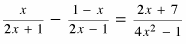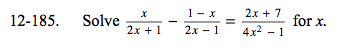### Home > A2C > Chapter 12 > Lesson 12.4.2 > Problem12-185

12-185.

Solvefor x. Homework Help ✎(2x + 1)(2x − 1) = 4x2 − 1

Multiply both sides by the least common multiple and simplify.

Set the equation equal to zero and factor, then you can use the Zero Product Property.

x = 2 or x = −1